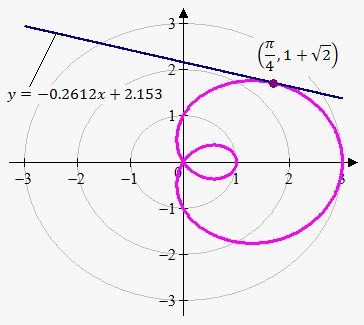# Tangent Line in Polar Coordinates

## Related calculator: Tangent Line Calculator

Let's see how to derive equation of tangent line when we are given equation of curve r=f(theta) in polar coordinates.

We will proceed in the same fashion as with tangent lines to parametric curves, because polar coordinates in some sense similar to parametric curves.

We know that x=rcos(theta) and y=rsin(theta).

Since we are given that r=f(theta) we should treat r as a function of theta.

To find slope of tangent line, we need to find (dy)/(dx) but in terms of r and theta. This can be easily done with chain rule:

(dy)/(dx)=(dy)/(dtheta)*(dtheta)/(dx)=(dy)/(dtheta)*1/((dx)/(dtheta)).

We have that (dy)/(dtheta)=(dr)/(dtheta)sin(theta)+rcos(theta) and (dx)/(dtheta)=(dr)/(dtheta)cos(theta)-rsin(theta).

So, we obtained following fact.

Fact. Slope of tangent line in polar coordinates is (dy)/(dx)=((dr)/(dtheta)sin(theta)+rcos(theta))/((dr)/(dtheta)cos(theta)-rsin(theta)).

Example. Find equation of tangent line to r=1+2cos(theta) at theta=pi/4.We have that (dr)/(dtheta)=-2sin(theta) , so

(dy)/(dx)=(-2sin(theta)sin(theta)+(1+2cos(theta))cos(theta))/(-2sin(theta)cos(theta)-(1+2cos(theta))sin(theta))=

=(cos(theta)+2cos(2theta))/(-sin(theta)-2sin(2theta)).

So, slope of tangent line at point theta=pi/4 is m=(dy)/(dx)|_(theta=pi/4)=(cos(pi/4)+2cos(pi/2))/(-sin(pi/4)-2sin(pi/2))=1/7(1-2sqrt(2)).

Now we need corresponding x and y coordinates when theta=pi/4:

x=rcos(theta)=(1+2cos(theta))cos(theta)=(1+2cos(pi/4))cos(pi/4)=(1+sqrt(2))/sqrt(2).

y=rsin(theta)=(1+2cos(theta))sin(theta)=(1+2cos(pi/4))sin(pi/4)=(1+sqrt(2))/sqrt(2).

So, equation of tangent line that passes through point theta=pi/4 is y=1/7(1-2sqrt(2))(x-(1+sqrt(2))/sqrt(2))+(1+sqrt(2))/sqrt(2) or approximately y=-0.2612x+2.153.

As with parametric curves there are curves that have several tangent line at one point. For example, in above example, such point is (0,0). The corresponding value(s) of theta we can find by solving equation 1+2cos(theta)=0. This gives two solutions on interval [0,2pi]: (2pi)/3 and (4pi)/3, so there will be two tangent lines at (0,0).

Similarly to parametric curves, curve in polar coordinates has

• horizontal tangent when (dy)/(dtheta)=0 (provided that (dx)/(dtheta)!=0).
• vertical tangent when (dx)/(dtheta)=0 (provided that (dy)/(dtheta)!=0).